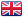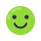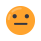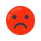info_outline

This is a restricted product and it cannot be activated and played in Australia.

For this product you will receive a link to an external platform instead of a regular CD Key.

Region:GLOBAL (Exceptions - see the note above)
Languages:Stock: In stock
info_outline

Dedicated 24/7 support, One-Contact Resolution and much more

## local_offer Offers

SupplierRatingOrders Price
Sort by:Playtime
99.63%
•Positive 571101
•Neutral 1516
•Negative 2093

POSITIVE FEEDBACK
392831 € 21.68Plyxa
98.97%
•Positive 78060
•Neutral 655
•Negative 810

POSITIVE FEEDBACK
39453 € 21.69

## local_offer Offers

Playtime (392831)
99.63%
•Positive 571101
•Neutral 1516
•Negative 2093

POSITIVE FEEDBACK
€ 21.68
Plyxa (39453)
98.97%
•Positive 78060
•Neutral 655
•Negative 810

POSITIVE FEEDBACK
€ 21.69

## description Description and requirements

How to Activate:

• Make sure to choose the same country as your store country found in Steam account details.
• After you accept the friend request, it will send you your purchased game as a gift.
##### Features:
• Dedicated Servers
• Competitive Search And Destroy game mode
• Casual fast-paced game modes
• Bots
• Matchmaking
• Offline/Practice mode
• Voice Chat

The gift cannot be accepted into an inventory and sent to a different account. If you want to gift the product - please sent them the link you receive directly.

Discover the best Game Key and Steam Gifts offers, compare prices to download and play Pavlov VR at the best cost. Compare the Steam Gift price from suppliers all around the world. Activate CD Keys on your Steam client to download the games and play in multiplayer or singleplayer.

GAMIVO is a platform to find, compare and buy digital game keys. Only verified and trusted suppliers can sell digital goods on GAMIVO.

How to activate Steam Gift products?

Open the received CD key link and login there using your Steam account. Accept a friend request using the Steam application. You will now receive the purchased game as a gift. The CD key has been activated and added to your Steam library. You can play now!

## descriptionDescription and requirementskeyboard_arrow_down

How to Activate:

• Make sure to choose the same country as your store country found in Steam account details.
• After you accept the friend request, it will send you your purchased game as a gift.
##### Features:
• Dedicated Servers
• Competitive Search And Destroy game mode
• Casual fast-paced game modes
• Bots
• Matchmaking
• Offline/Practice mode
• Voice Chat

## message Reviews

### Pavlov VR is a fun game

Pavlov vr is a fun vr game and is a must have :))))))))))))))))))))))))))))))))))))))))))))))))))))))))))))))))))))))))))))))))))))))))))))))))))))))))))))))))))))))))))))))))))))))))))))))))))))))))))))))))))))))))))))))))))))))))))))))))))))))))))))))))))))))))))))))))))))))))))))))))))))))))))))))))))))))))))))))))))))))))))))))))))))))))))))))))))))))))))))))))))))))))))))))))))))))))))))))))))))))))))))))))))))))))))))))))))))))))))))))))))))))))))))))))))))))))
(0 of 0 users found this helpful)

### BEST VR GAME!

I've racked on 291 hours, and I still play
This is the best VR game, to me. So many different gamer modes, that I never get bored. Only reason I turn it off, is to go to sleep, if I even do sleep at all X-D

Jailbreak mode is the best. Now supports something like 20 players, in one server :-o
Battle Royal is pretty fun too.

Guns are pretty realistic. I am currently unable to be in possession of firearms, so this fixes that itch X-]
(0 of 0 users found this helpful)